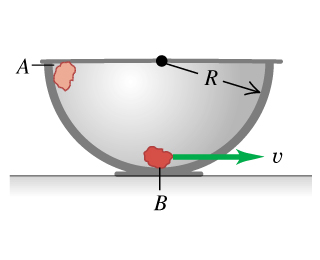# Problem: A small rock with mass 0.22 kg is released from rest at point exttip{A}{A}, which is at the top edge of a large, hemispherical bowl with radius exttip{R}{R} = 0.52 m (the figure ). Assume that the size of the rock is small compared to R, so that the rock can be treated as a particle, and assume that the rock slides rather than rolls. The work done by friction on the rock when it moves from point exttip{A}{A} to point exttip{B}{B} at the bottom of the bowl has magnitude 0.22 J.Between points exttip{A}{A} and exttip{B}{B}, how much work is done on the rock by the normal force?What is the speed of the rock as it reaches point exttip{B}{B}?Of the three forces acting on the rock as it slides down the bowl, which (if any) are constant and which are not? Explain.Just as the rock reaches point exttip{B}{B}, what is the normal force on it due to the bottom of the bowl?Between points exttip{A}{A} and exttip{B}{B}, how much work is done on the rock by gravity?

⚠️Our tutors found the solution shown to be helpful for the problem you're searching for. We don't have the exact solution yet.

###### Problem Details

A small rock with mass 0.22 kg is released from rest at point , which is at the top edge of a large, hemispherical bowl with radius = 0.52 m (the figure). Assume that the size of the rock is small compared to R, so that the rock can be treated as a particle, and assume that the rock slides rather than rolls. The work done by friction on the rock when it moves from point to point at the bottom of the bowl has magnitude 0.22 J.

Between points and , how much work is done on the rock by the normal force?

What is the speed of the rock as it reaches point ?

Of the three forces acting on the rock as it slides down the bowl, which (if any) are constant and which are not? Explain.

Just as the rock reaches point , what is the normal force on it due to the bottom of the bowl?

Between points and , how much work is done on the rock by gravity?